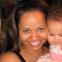Java Games: Flashcards, matching, concentration, and word search.# Verbal Expressions

Translate verbal expressions into algebraic expressions.

AB
Three more than twice a number2n + 3
The difference of 3 and a number squared3 - n^2
Three less than the square of a numbern^2 - 3
The sum of 3 and twice a number3 + 2n
The quotient of a number and 3n/3
The product of a number and 33n
The difference of a number and 3n - 3
3 less than the quotient of 4 and a number4/n - 3
The difference of 3 and the quotient of 4 and a number3 - 4/n
The quotient of 3 and a number3/n
5 less than the product of 6 and a number6n - 5
The difference of 5 and the product of 6 and a number5 - 6n
The sum of the quotient of 3 and a number and 4(3/n + 4)
The sum of the difference of 6 and a number and 3(6 - n) + 3
The square of a numbern^2
Twice a number2nMrs. Foulke Benjamin Syms Middle School Hampton, VA

 This activity was created by a Quia Web subscriber. Learn more about QuiaCreate your own activities